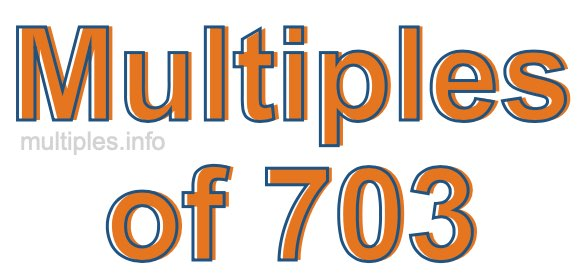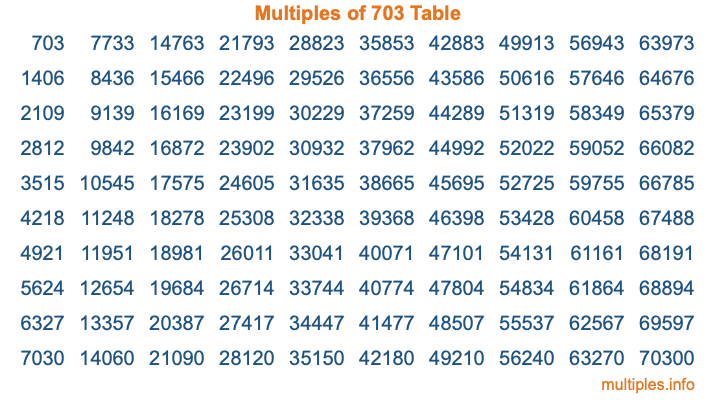Multiples of 703Welcome to the Multiples of 703 page. Here we will first teach you everything you will ever need to know about the multiples of 703, and then give you a study guide summary of everything we taught you to make sure you remember it all. Use this page to look up facts and learn information about the multiples of 703. This page will make you a multiples of seven hundred three expert!

Definition of Multiples of 703
Multiples of 703 are all the numbers that when divided by 703 equal an integer. Each of the multiples of 703 are called a multiple. A multiple of 703 is created by multiplying 703 by an integer.

Therefore, to create a list of multiples of 703, you start with 1 multiplied by 703, then 2 multiplied by 703, then 3 multiplied by 703, and so on for as long as you want. Thus, the list of the first five multiples of 703 is 703, 1406, 2109, 2812, and 3515. To see a larger list of multiples of 703, see the printable image of Multiples of 703 further down on this page. We also have a category where you can choose any nth multiple of 703.

Multiples of 703 Checker
The Multiples of 703 Checker below checks to see if any number of your choice is a multiple of 703. In other words, it checks to see if there is any number (integer) that when multiplied by 703 will equal your number. To do that, we divide your number by 703. If the the quotient is an integer, then your number is a multiple of 703.

Is  a multiple of 703?

Least Common Multiple of 703 and ...
A Least Common Multiple (LCM) is the lowest multiple that two or more numbers have in common. This is also called the smallest common multiple or lowest common multiple and is useful to know when you are adding our subtracting fractions. Enter one or more numbers below (703 is already entered) to find the LCM.

Check out our LCM Calculator if you need more details about the Least Common Multiple or if you need the LCM for different numbers for adding and subtraction fractions.

nth Multiple of 703
As we stated above, 703 is the first multiple of 703, 1406 is the second multiple of 703, 2109 is the third multiple of 703, and so on. Enter a number below to find the nth multiple of 703.

th multiple of 703

Multiples of 703 vs Factors of 703
703 is a multiple of 703 and a factor of 703, but that is where the similarities end. All postive multiples of 703 are 703 or greater than 703. All positive factors of 703 are 703 or less than 703.

Below is the beginning list of multiples of 703 and the factors of 703 so you can compare:

Multiples of 703: 703, 1406, 2109, 2812, 3515, etc.

Factors of 703: 1, 19, 37, 703

As you can see, the multiples of 703 are all the numbers that you can divide by 703 to get a whole number. The factors of 703, on the other hand, are all the whole numbers that you can multiply by another whole number to get 703.

It's also interesting to note that if a number (x) is a factor of 703, then 703 will also be a multiple of that number (x).

Multiples of 703 vs Divisors of 703
The divisors of 703 are all the integers that 703 can be divided by evenly. Below is a list of the divisors of 703.

Divisors of 703: 1, 19, 37, 703

The interesting thing to note here is that if you take any multiple of 703 and divide it by a divisor of 703, you will see that the quotient is an integer.

Multiples of 703 Table
Below is an image of the first 100 multiples of 703 in a table. The table is in chronological order, column by column. The first column has the first ten multiples of 703, the second column has the next ten multiples of 703, and so on.The Multiples of 703 Table is also referred to as the 703 Times Table or Times Table of 703. You are welcome to print out our table for your studies.

Negative Multiples of 703
Although not often discussed or needed in math, it is worth mentioning that you can make a list of negative multiples of 703 by multiplying 703 by -1, then by -2, then by -3, and so on, to get the following list of negative multiples of 703:

-703, -1406, -2109, -2812, -3515, etc.

Multiples of 703 Summary
Below is a summary of important Multiples of 703 facts that we have discussed on this page. To retain the knowledge on this page, we recommend that you read through the summary and explain to yourself or a study partner why they hold true.

There are an infinite number of multiples of 703.

A multiple of 703 divided by 703 will equal a whole number.

703 divided by a factor of 703 equals a divisor of 703.

The nth multiple of 703 is n times 703.

The largest factor of 703 is equal to the first positive multiple of 703.

703 is a multiple of every factor of 703.

703 is a multiple of 703.

A multiple of 703 divided by a divisor of 703 equals an integer.

703 divided by a divisor of 703 equals a factor of 703.

Any integer times 703 will equal a multiple of 703.

Multiples of a Number
Here you can get the multiples of another number, all with the same attention to detail as we did for multiples of 703 on this page.

Multiples of
Multiples of 704
Did you find our page about multiples of seven hundred three educational? Do you want more knowledge? Check out the multiples of the next number on our list!